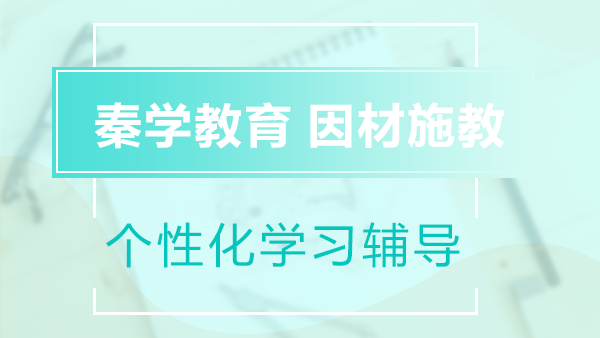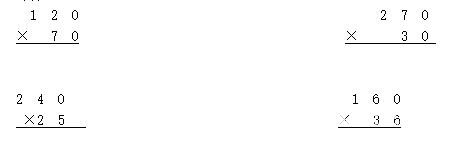2017小学四年级上册同步练习(8)，坚持就是胜利!小新2017.08.16

小学四年级的数学，开始讲究技巧，因此好多孩子都被家长逼着去上思维数学课。殊不知，这样不仅导致孩子没有时间复习基础，也让孩子没有心理负担加重。秦学教育小编建议对于基础不是很好的孩子，只要多做练习，多复习巩固，一样是可以学好数学的。一起来做做较新人教版小学四年级上册数学同步练习题吧~练习八

基础达标：

一、 口算

70×90=　　　　　　31×40=　　　　　　　　　24×20=　　　　　　　40×80=

5×60=　　　　　　 150×3= 140×5= 300×20=

80×12=　　　　　　310×4=　　　　　　　　　32×40=　　　　　　　250×4=

二、 计算三、 列竖式计算，并用计算器验算

340×24=　　　　　　　　　　　12×520=　　　　　　　　　　　309×20=

41×80=　　　　　　　　　　　304×30=　　　　　　　　　　　　170×32=

四、 填空

⒈　自行车的速度可达每小时15千米，可以写作：(　　　　　　　　　　　　　　　　)。

⒉　人步行的速度可达每分钟30米，可以写作：(　　　　　　　　　　　　　　　　　)。

⒊　光在空气中的速度可达每秒钟30000千米，可以写作：(　　　　　　　　　　　　)。

⒋　(　　　　　)×(　　　　　)=路程，路程÷(　　　　　)=(　　　　　)。

拓展创新：

五、 解决问题

⒈　飞行员们要每天训练3小时飞行，训练飞机飞行的速度大约是760千米/时，飞行员们每天飞行多少千米?

⒉　李红同学和父母一起坐车去南京游玩，去的时候汽车的速度是80千米/时，行了3小时，回来的时候行了4小时。

⑴　李红同学家到南京的路程大约是多少千米?

⑵　从南京回来的时候，汽车的速度大约是多少?

⑶　如果这辆汽车的速度是40千米/时，那么从李红家出发，几小时后到达南京?

综合探索：

六、灵感闪现

用0，7，6，2，8组成三位数乘两位数的乘法算式，你能写出几个?你能写出乘积较大的算式吗?

18066521615加入家长交流圈，添加微信号18066521615+年级学科资料